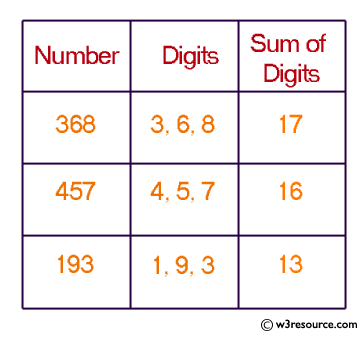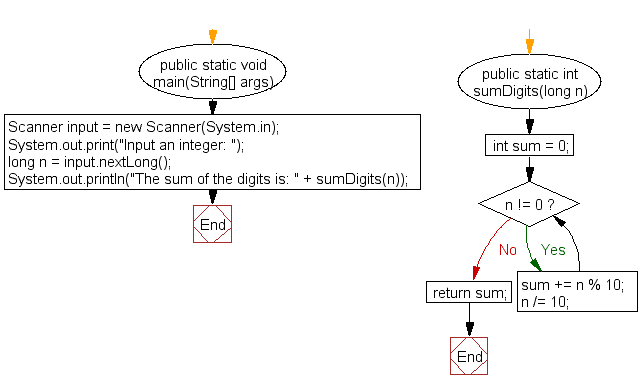﻿ Java exercises: Compute the sum of the digits of an integer - w3resource# Java Exercises: Compute the sum of the digits of an integer

## Java Basic: Exercise-33 with Solution

Write a Java program and compute the sum of the digits of an integer.

Test Data:
Input an intger: 25

Pictorial Presentation:Sample Solution:

Java Code:

``````import java.util.Scanner;
public class Exercise33 {
public static void main(String[] args) {
Scanner input = new Scanner(System.in);
System.out.print("Input an integer: ");
long n = input.nextLong();
System.out.println("The sum of the digits is: " + sumDigits(n));

}

public static int sumDigits(long n) {
int sum = 0;
while (n != 0) {
sum += n % 10;
n /= 10;
}
return sum;
}
}
```
```

Sample Output:

```Input an intger: 25
The sum of the digits is: 7
```

Flowchart:Java Code Editor:

What is the difficulty level of this exercise?

Test your Programming skills with w3resource's quiz.

﻿

## Java: Tips of the Day

getEnumMap

Converts to enum to Map where key is the name and value is Enum itself.

```public static <E extends Enum<E>> Map<String, E> getEnumMap(final Class<E> enumClass) {
return Arrays.stream(enumClass.getEnumConstants())
.collect(Collectors.toMap(Enum::name, Function.identity()));
}
```

Ref: https://bit.ly/3xXcFZt